# Linear function

(diff) ← Older revision | Latest revision (diff) | Newer revision → (diff)

A function of the form. The main property of a linear function is: The increment of the function is proportional to the increment of the argument. Graphically a linear function is represented by a straight line.

A linear function invariablesis a function of the form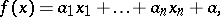whereandare certain fixed numbers. The domain of definition of a linear function is the whole-dimensional space of the variables, real or complex. If, the linear function is called a homogeneous, or linear, form.

If all variablesand coefficientsare real (complex) numbers, then the graph of the linear function in the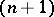-dimensional (complex) space of the variables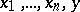is the (complex)-dimensional hyperplane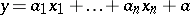, in particular, forit is a straight line in the plane (respectively, a complex plane in two-dimensional complex space).

The term "linear function" , or, more precisely, homogeneous linear function, is often used for a linear mapping of a vector spaceover a fieldinto this field, that is, for a mappingsuch that for any elementsand any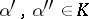,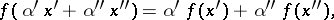and in this case instead of the term "linear function" one also uses the terms linear functional and linear form.

How to Cite This Entry:
Linear function. Encyclopedia of Mathematics. URL: http://encyclopediaofmath.org/index.php?title=Linear_function&oldid=19167
This article was adapted from an original article by L.D. Kudryavtsev (originator), which appeared in Encyclopedia of Mathematics - ISBN 1402006098. See original article